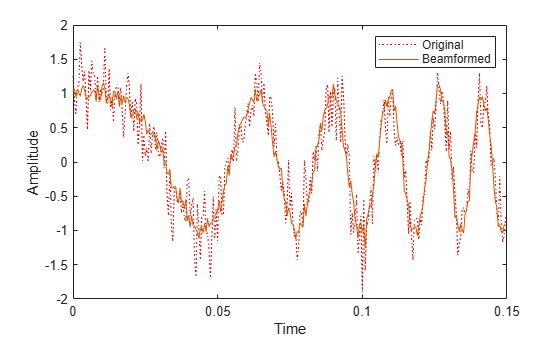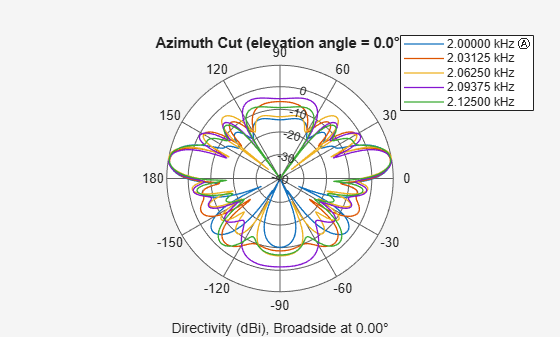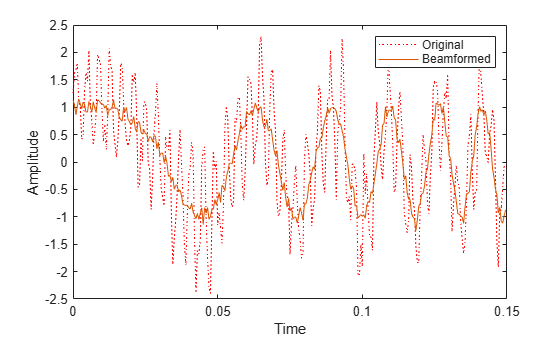# phased.SubbandMVDRBeamformer

Wideband minimum-variance distortionless-response beamformer

## Description

The `phased.SubbandMVDRBeamformer` System object™ implements a wideband minimum variance distortionless response beamformer (MVDR) based on the subband processing technique. This type of beamformer is also called a Capon beamformer.

To beamform signals arriving at an array:

1. Create the `phased.SubbandMVDRBeamformer` object and set its properties.

2. Call the object with arguments, as if it were a function.

## Creation

### Syntax

``beamformer = phased.SubbandMVDRBeamformer``
``beamformer = phased.SubbandMVDRBeamformer(Name,Value)``

### Description

````beamformer = phased.SubbandMVDRBeamformer` creates a subband MVDR beamformer System object, `beamformer`. The object performs subband MVDR beamforming on the received signal.```
````beamformer = phased.SubbandMVDRBeamformer(Name,Value)` creates a subband MVDR beamformer System object, `beamformer`, with each specified property `Name` set to the specified `Value`. You can specify additional name-value pair arguments in any order as `Name1`,`Value1`,...,`NameN`,`ValueN`.Example: ```beamformer = phased.SubbandMVDRBeamformer('SensorArray',phased.URA('Size',[5 5]),'OperatingFrequency',500e6)``` sets the sensor array to a 5-by-5 uniform rectangular array (URA) with all other default URA property values. The beamformer has an operating frequency of 500 MHz.```

## Properties

expand all

Unless otherwise indicated, properties are nontunable, which means you cannot change their values after calling the object. Objects lock when you call them, and the `release` function unlocks them.

If a property is tunable, you can change its value at any time.

Sensor array, specified as an array System object belonging to Phased Array System Toolbox. The sensor array can contain subarrays.

Example: `phased.URA`

Signal propagation speed, specified as a real-valued positive scalar. Units are in meters per second. The default propagation speed is the value returned by `physconst('LightSpeed')`.

Example: `3e8`

Data Types: `single` | `double`

Operating frequency, specified as a positive scalar. Units are in Hz.

Example: `1e9`

Data Types: `single` | `double`

Sample rate of signal, specified as a positive scalar. Units are in Hz. The System object uses this quantity to calculate the propagation delay in units of samples.

Example: `1e6`

Data Types: `single` | `double`

Number of processing subbands, specified as a positive integer.

Example: `128`

Data Types: `double`

Source of beamforming direction, specified as `'Property'` or ```'Input port'```. Specify whether the beamforming direction comes from the `Direction` property of this object or from the input argument, `ANG`. Values of this property are:

 `'Property'` Specify the beamforming direction using the `Direction` property. `'Input port'` Specify the beamforming direction using the input argument, `ANG`.

Data Types: `char`

Beamforming directions, specified as a real-valued 2-by-1 vector or a real-valued 2-by-L matrix. For a matrix, each column specifies a different beamforming direction. Each column has the form `[AzimuthAngle;ElevationAngle]`. Azimuth angles must lie between –180° and 180° and elevation angles must lie between –90° and 90°. All angles are defined with respect to the local coordinate system of the array. Units are in degrees.

Example: `[40;30]`

#### Dependencies

To enable this property, set the `DirectionSource` property to `'Property'`.

Data Types: `single` | `double`

Diagonal loading factor, specified as a nonnegative scalar. Diagonal loading is a technique used to achieve robust beamforming performance, especially when the sample size is small. A small sample size can lead to an inaccurate estimate of the covariance matrix. Diagonal loading also provides robustness due to steering vector errors. The diagonal loading technique adds a positive scalar multiple of the identity matrix to the sample covariance matrix.

Tunable: Yes

Data Types: `single` | `double`

Enable training data input, specified as `false` or `true`. When you set this property to `true`, use the training data input argument, `XT`, when running the object. Set this property to `false` to use the input data, `X`, as the training data.

Data Types: `logical`

Enable the output of beamforming weights, specified as `false` or `true`. To obtain the beamforming weights, set this property to `true` and use the corresponding output argument, `W`. If you do not want to obtain the weights, set this property to `false`.

Data Types: `logical`

Option to enable output of subband center frequencies, specified as either `true` or `false`. To obtain the subband center frequencies, set this property to `true` and use the corresponding output argument `FREQS` when calling the object.

Data Types: `logical`

## Usage

### Syntax

``Y = beamformer(X)``
``Y = beamformer(X,XT)``
``Y = beamformer(X,ANG)``
``````[Y,W] = beamformer(___)``````
``[Y,FREQS] = beamformer(___)``
``````[Y,W,FREQS] = beamformer(X,XT,ANG)``````

### Description

````Y = beamformer(X)` performs wideband MVDR beamforming on the input, `X`, and returns the beamformed output in `Y`. This syntax uses `X` for training samples to calculate the beamforming weights. Use the Direction property to specify the beamforming direction.```
````Y = beamformer(X,XT)` uses `XT` for training samples to calculate the beamforming weights.```
````Y = beamformer(X,ANG)` uses `ANG` as the beamforming direction. This syntax applies when you set the DirectionSource property to `'Input port'`.```
``````[Y,W] = beamformer(___)``` returns the beamforming weights, `W`. This syntax applies when you set the WeightsOutputPort property to `true`. ```
````[Y,FREQS] = beamformer(___)` returns the center frequencies of the subbands, `FREQS`. This syntax applies when you set the SubbandsOutputPort property to `true`.```

example

```You can combine optional input arguments when you set their enabling properties. Optional input arguments must be listed in the same order as their enabling properties. For example, ```[Y,W,FREQS] = beamformer(X,XT,ANG)``` is valid when you specify TrainingInputPort as `true` and set DirectionSource to `'Input port'`.```

### Input Arguments

expand all

Wideband input signal, specified as an M-by-N matrix, where N is the number of array elements. M is the number of samples in the data. If the sensor array consists of subarrays, N is then the number of subarrays.

The size of the first dimension of the input matrix can vary to simulate a changing signal length. A size change can occur, for example, in the case of a pulse waveform with variable pulse repetition frequency.

If you set the TrainingInputPort to `false`, then the object uses `X` as training data. In this case, the dimension M must be greater than N×NB. where NB is the number of subbands specified in the NumSubbands.

If you set TrainingInputPort to `true`, use the `XT` argument to supply training data. In this case, the dimension M can be any positive integer.

Example: `[1,1;j,1;0.5,0]`

Data Types: `single` | `double`
Complex Number Support: Yes

Wideband training samples, specified as a P-by-N matrix where N is the number of elements. If the sensor array consists of subarrays, then N represents the number of subarrays.

The size of the first dimension of the input matrix can vary to simulate a changing signal length. A size change can occur, for example, in the case of a pulse waveform with variable pulse repetition frequency.

This argument applies when you set TrainingInputPort to `true`. The dimension P is the number of samples in the training data. P must be larger than N×NB, where NB is the number of subbands specified in the NumSubbands property.

Example: `FT = [1,1;j,1;0.5,0]`

Data Types: `single` | `double`
Complex Number Support: Yes

Beamforming direction, specified as a 2-by-L real-valued matrix, where L is the number of beamforming directions. This argument applies only when you set the DirectionSource property to `'Input port'`. Each column takes the form of `[AzimuthAngle;ElevationAngle]`. Angle units are in degrees. The azimuth angle must lie between –180° and 180°. The elevation angle must lie between –90° and 90°. Angles are defined with respect to the local coordinate system of the array.

Example: `[40 30;0 10]`

Data Types: `single` | `double`

### Output Arguments

expand all

Beamformed output, returned as an M-by-L complex-valued matrix. The quantity M is the number of signal samples and L is the number of beamforming directions specified in the `ANG` argument.

Beamforming weights, returned as an N-by-K-by-L complex-valued matrix. The quantity N is the number of sensor elements or subarrays and K is the number of subbands specified by the NumSubbands property. The quantity L is the number of beamforming directions. Each column of `W` contains the narrowband beamforming weights used in the corresponding subband for the corresponding directions.

#### Dependencies

To return this output, set the WeightsOutputPort property to `true`.

Data Types: `single` | `double`

Center frequencies of subbands, returned as a K-by-1 real-valued column vector. The quantity K is the number of subbands specified by the NumSubbands property.

#### Dependencies

To return this output, set the SubbandsOutputPort property to `true`.

Data Types: `single` | `double`

## Object Functions

To use an object function, specify the System object as the first input argument. For example, to release system resources of a System object named `obj`, use this syntax:

`release(obj)`

expand all

 `step` Run System object algorithm `release` Release resources and allow changes to System object property values and input characteristics `reset` Reset internal states of System object

## Examples

collapse all

Apply subband MVDR beamforming to an underwater acoustic 11-element ULA. The incident angle of the signal is $1{0}^{\circ }$ azimuth and ${0}^{\circ }$ elevation. The signal is an FM chirp having a bandwidth of 1 kHz. The speed of sound is 1500 m/s.

Simulate signal

```array = phased.ULA('NumElements',11,'ElementSpacing',0.3); fs = 2e3; carrierFreq = 2000; t = (0:1/fs:2)'; sig = chirp(t,0,2,fs/2); c = 1500; collector = phased.WidebandCollector('Sensor',array,'PropagationSpeed',c,... 'SampleRate',fs,'ModulatedInput',true,... 'CarrierFrequency',carrierFreq); incidentAngle = [10;0]; sig1 = collector(sig,incidentAngle); noise = 0.3*(randn(size(sig1)) + 1j*randn(size(sig1))); rx = sig1 + noise;```

Apply MVDR beamforming

```beamformer = phased.SubbandMVDRBeamformer('SensorArray',array,... 'Direction',incidentAngle,'OperatingFrequency',carrierFreq,... 'PropagationSpeed',c,'SampleRate',fs,'TrainingInputPort',true, ... 'SubbandsOutputPort',true,'WeightsOutputPort',true); [y,w,subbandfreq] = beamformer(rx, noise);```

Plot the signal that is input to the middle sensor (channel 6) vs the beamformer output.

```plot(t(1:300),real(rx(1:300,6)),'r:',t(1:300),real(y(1:300))) xlabel('Time') ylabel('Amplitude') legend('Original','Beamformed');```Plot array response

Plot the response pattern for five bands

```pattern(array,subbandfreq(1:5).',-180:180,0,... 'PropagationSpeed',c,'Weights',w(:,1:5));```Apply subband MVDR beamforming to an underwater acoustic 11-element ULA. Beamform the arriving signals to optimize the gain of a linear FM chirp signal arriving from 0 degrees azimuth and 0 degrees elevation. The signal has a bandwidth of 2.0 kHz. In addition, there unit amplitude 2.250 kHz interfering sine wave arriving from 28 degrees azimuth and 0 degrees elevation. Show how the MVDR beamformer nulls the interfering signal. Display the array pattern for several frequencies in the neighborhood of 2.250 kHz. The speed of sound is 1500 meters/sec.

Simulate Arriving Signal and Noise

```array = phased.ULA('NumElements',11,'ElementSpacing',0.3); fs = 2000; carrierFreq = 2000; t = (0:1/fs:2)'; sig = chirp(t,0,2,fs/2); c = 1500; collector = phased.WidebandCollector('Sensor',array,'PropagationSpeed',c,... 'SampleRate',fs,'ModulatedInput',true,... 'CarrierFrequency',carrierFreq); incidentAngle = [0;0]; sig1 = collector(sig,incidentAngle); noise = 0.3*(randn(size(sig1)) + 1j*randn(size(sig1)));```

Simulate Interfering Signal

Combine both the desired and interfering signals.

```fint = 250; sigint = sin(2*pi*fint*t); interfangle = [28;0]; sigint1 = collector(sigint,interfangle); rx = sig1 + sigint1 + noise;```

Apply MVDR Beamforming

Use the combined noise and interfering signal as training data.

```beamformer = phased.SubbandMVDRBeamformer('SensorArray',array,... 'Direction',incidentAngle,'OperatingFrequency',carrierFreq,... 'PropagationSpeed',c,'SampleRate',fs,'TrainingInputPort',true,... 'NumSubbands',64,... 'SubbandsOutputPort',true,'WeightsOutputPort',true); [y,w,subbandfreq] = beamformer(rx,sigint1 + noise); tidx = [1:300]; plot(t(tidx),real(rx(tidx,6)),'r:',t(tidx),real(y(tidx))) xlabel('Time') ylabel('Amplitude') legend('Original','Beamformed')```Plot Array Response Showing Beampattern Null

Plot the response pattern for five bands near 2.250 kHz.

```fdx = [5,7,9,11,13]; pattern(array,subbandfreq(fdx).',-50:50,0,... 'PropagationSpeed',c,'Weights',w(:,fdx),... 'CoordinateSystem','rectangular');```The beamformer places a null at 28 degrees for the subband containing 2.250 kHz.